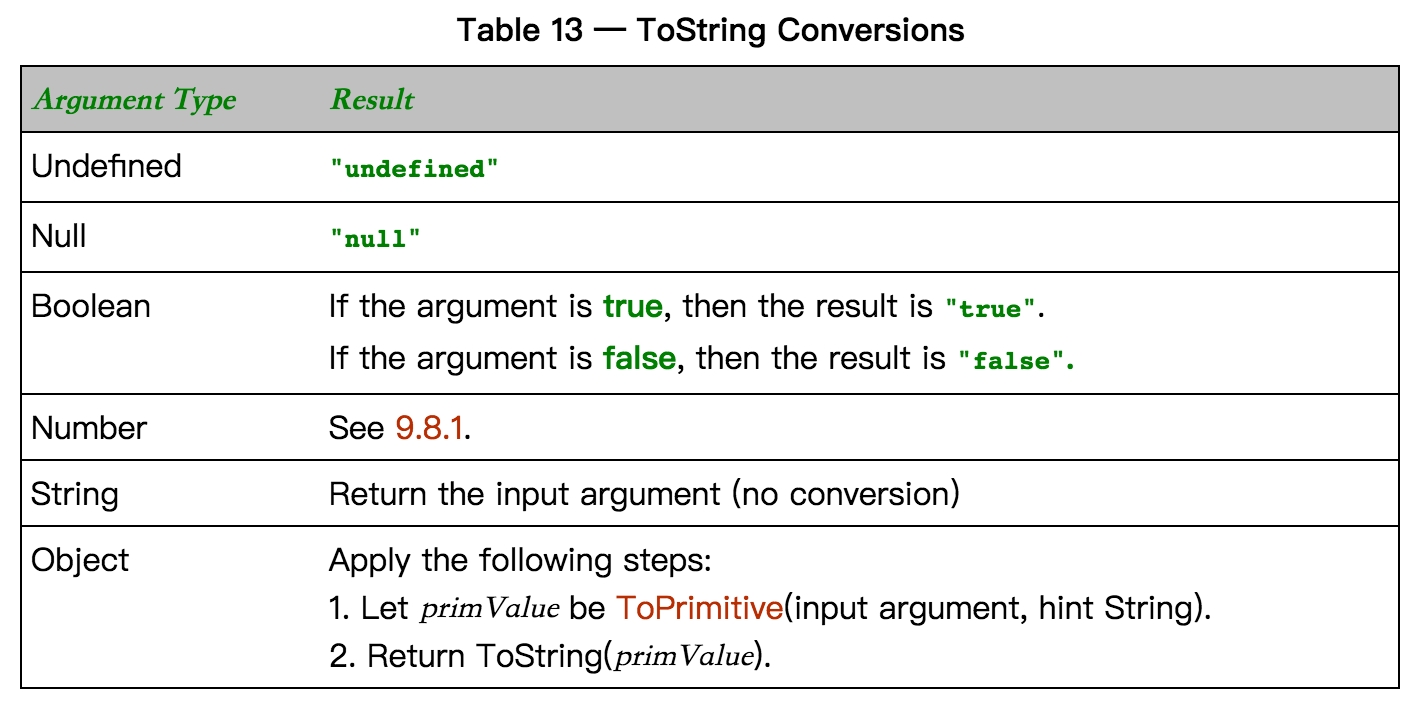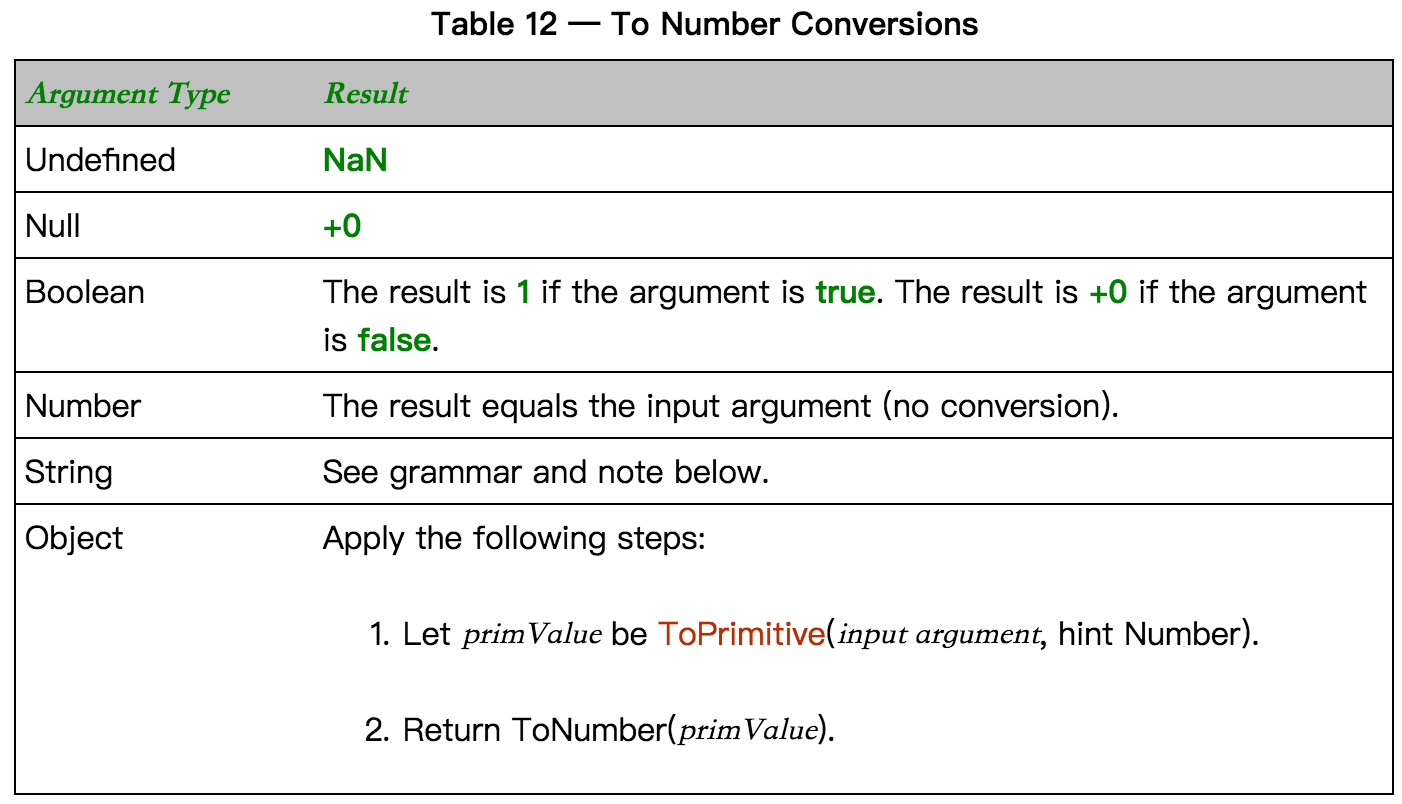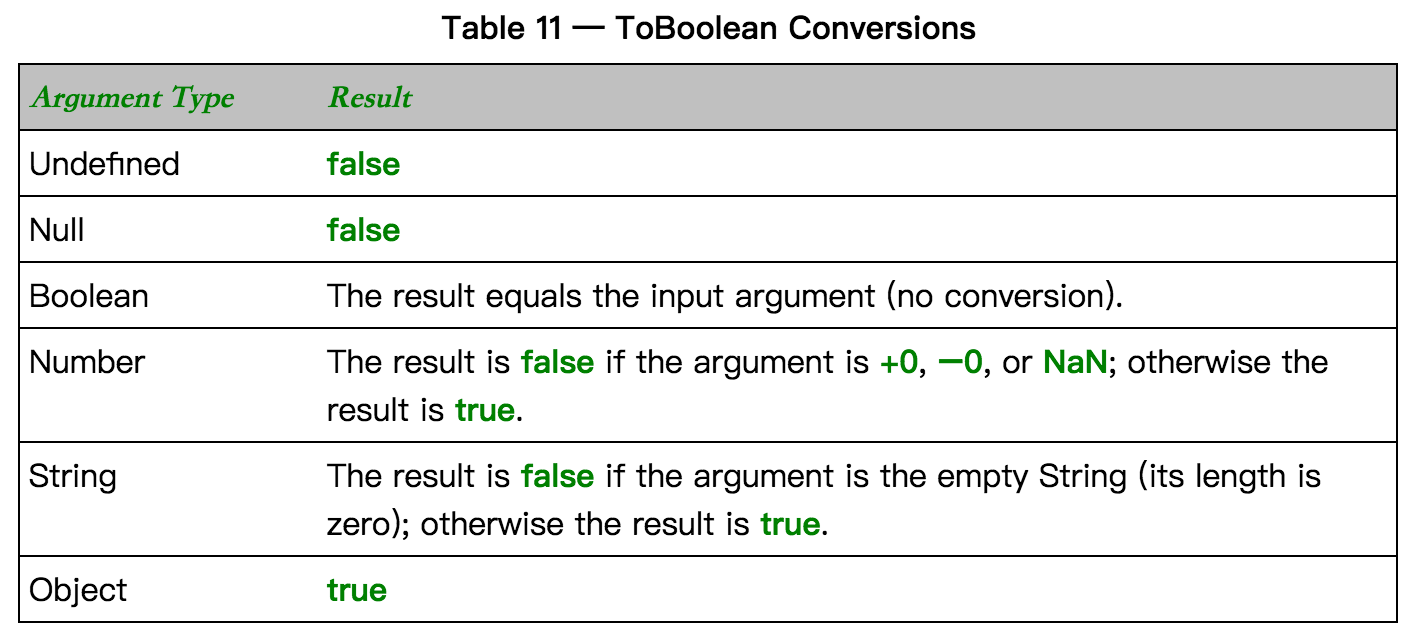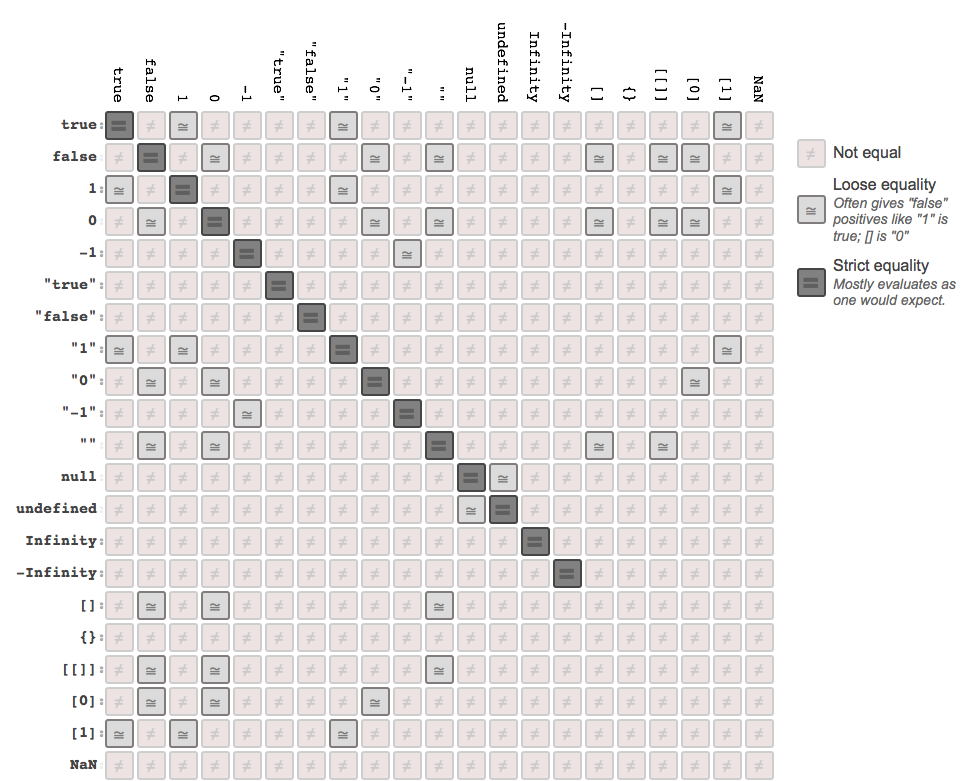# 你懂 JavaScript 嗎？#8 強制轉型（Coercion）• 強制轉型（coercion）分為兩種，分別是「明確的」強制轉型（explicit coercion）和「隱含的」強制轉型（implicit coercion），只要是程式碼中刻意寫出來的型別轉換的動作，就是明確的強制轉型；反之，在程式碼中沒有明確指出要轉換型別卻轉型的，就是隱含的強制轉型。
• 明確的強制轉型規則與範例說明。
• 隱含的強制轉型規則與範例說明。
• Symbol 的強制轉型的規則與範例說明。
• 隱含的強制轉型的心酸血淚？各種令人崩潰的範例
• 抽象的關係式比較

## 前言

``````var a = 42;
var b = String(a); // 明確的強制轉型
var c = a + ''; // 隱含的強制轉型

b; // '42'
c; // '42'
``````

## 抽象的值運算

「抽象的值運算」指的是「內部限定的運算」，意即這是 JavaScript 引擎在背後偷偷幫我們做的工作。在這個部份會來探討 ToStringToNumberToBooleanToPrimitive 這幾個抽象的值運算，來看看到底在強制轉型時背地裡做了什麼好事。### ToString

• undefined -> `'undefined'`
• null -> `'null'`
• boolean 的 true -> `'true'`，false -> `'false'`
• 在數字方面，非常大或非常小的數字以指數呈現，例如：`'1.23e21'`
• 物件
• 若有定義其 `toString` 方法，則會以它自己的 `toString` 方法所產生的結果為優先，例如，陣列有自己定義的 `toString` 方法，因此 `[1,2,3].toString()` 會得到 `"1,2,3"`
• 若沒有定義 `toString` 方法，則回傳內部的屬性 `[[Class]]` ，這是一個用來標記這個值是屬於物件的哪個子分類的標籤，例如：`({}).toString()` 會得到 `[object Object]`#### JSON 的字串化（JSON Stringification）

JSON 的字串化 `JSON.stringify` 將值序列化（serialize）為 JSON 字串，這個轉為 JSON 字串的過程與 ToString 規則有關，但並不等於強制轉型。

• 若為簡單值，即字串、數字、布林、null，則規則與 ToString 相同。這些能轉為 JSON 字串的值稱為是「JSON-safe」的值，意即只要對 JSON 來說是安全的（safe），就都能轉為 JSON 字串。
``````JSON.stringify(42); // "42"
JSON.stringify(true); // "true"
JSON.stringify(null); // "null"
JSON.stringify('Hello World'); // ""Hello World""，字串會在外面再包一層引號！
``````
• 無法轉為 JSON 字串的非法值有 undefined、function、symbol、具有循環參考（circular reference）的物件，由於它們無法轉為 JSON 字串，因此 `JSON.stringify` 會自動忽略這些非法值或丟出錯誤。又，若陣列中某個元素的值為非法值則會自動以 null 取代；若物件中的其中一個屬性為非法值，則會排除這個屬性。
• 若為物件且有定義 `toJSON` 方法則會優先呼叫此方法，並依此方法之回傳值作為序列化的結果。因此，若試圖 JSON 字串化一個含有非法值的物件，應定義其 `toJSON` 方法以回傳適當的 JSON-safe 的值。

``````JSON.stringify(undefined); // undefined，忽略非法值
JSON.stringify(function () {}); // undefined，忽略非法值
JSON.stringify(Symbol()); // undefined，忽略非法值
JSON.stringify([1, 2, 3, undefined]); // "[1,2,3,null]"，非法值以 null 取代
JSON.stringify({ a: 2, b: function () {} }); // "{"a":2}"，忽略非法屬性
``````

``````const a = { someProperty: 'Jack' };
const b = { anotherProperty: a };
a.b = b;

JSON.stringify(a); // Uncaught TypeError: Converting circular structure to JSON
JSON.stringify(b); // Uncaught TypeError: Converting circular structure to JSON
``````

``````const someObj = {
a: 2,
b: function () {}, // 非法!
toJSON: function () {
return {
a: 2, // 序列化過程只包含 a 屬性
};
},
};

JSON.stringify(someObj); // "{"a":2}"
``````

``````const a = {
someProperty: 'Jack',
toJSON: function () {
return {
prompt: 'Hello World',
};
},
};

const b = {
anotherProperty: a,
toJSON: function () {
return {
prompt: 'Hello World',
};
},
};

a.b = b;

// 序列化成功！不會被報錯了！
JSON.stringify(a); // "{"prompt":"Hello World"}"
JSON.stringify(b); // "{"prompt":"Hello World"}"
``````

• 取代器為陣列時，陣列內的元素為指定要包含的屬性名稱。如下，指定序列化過程中只需要包含 a 屬性。
``````const someObj = {
a: 2,
b: function () {},
};

JSON.stringify(someObj, ['a']); // "{"a":2}"
``````
• 取代器為函數時，函式是用來運算要回傳以做序列化的屬性的值。如下，指定除了 b 以外的屬性都要做序列化。
``````const someObj = {
a: 2,
b: function () {},
};

JSON.stringify(someObj, function (key, value) {
if (key !== 'b') {
return value;
}
});

// "{"a":2}"
``````

### ToNumber

• undefined -> NaN。
• null -> +0 即是 0。
• boolean 的 true -> 1，false -> +0 即是 0。
• string -> 數字或 NaN。
• object
• 若有定義其 `valueOf` 方法，則會優先使用 `valueOf` 取得其基本型別值。
• 若沒有定義 `valueOf` 方法，則會改用 `toString` 方法取得其基本型別值，再用 ToNumber 轉為數字。在這裡先簡化為 `Number(..)` 會來處理這一連串的流程即可。
• 注意，以 `Object.create(null)` 建立的 null 沒有 `valueOf``toString` 方法，因此在試圖轉為基本型別值的時候會出錯，丟出 TypeError。``````Number(undefined) // NaN
Number(null) // 0
Number(true) // 1
Number(false) // 0
Number('12345') // 12345
Number('Hello World') // NaN
Number({ name: 'Jack' }}) // NaN

const a = {
name: 'Apple',
valueOf: function() {
return '999'
}
}

Number(a) // 999
``````

### ToBoolean#### Falsy 值

• `""` 空字串
• 0, -0, NaN
• null
• undefined
• false

• `'Hello World'` 非空字串
• 42 非零的有效數字
• `[], [1, 2, 3]` 陣列，不管是不是空的
• `{}, { name: 'Jack' }` 物件，不管是不是空的
• `function foo() {}` 函式
• true

#### Falsy 物件

``````const a = new String('');
const b = new Number(0);
const c = new Boolean(false);

!!a; // true
!!b; // true
!!c; // true
``````

### ToPrimitive

• undefined -> undefined（基本型別值，不轉換）。
• null -> null（基本型別值，不轉換）。
• boolean -> boolean（基本型別值，不轉換）。
• number -> number（基本型別值，不轉換）。
• string -> string（基本型別值，不轉換）。
• object：使用 `[[DefaultValue]]` 內部方法，依照傳入的參數來決定要使用 toString 或 valueOf 取得基本型別值，看參考規格

## 明確的強制轉型（Explicit Coercion）

「明確的強制轉型」是指程式碼中刻意寫出來的明顯的型別轉換的動作。

### 明確的 Strings <–> Numbers

#### 方法一：使用內建函式 `String(..)` 與 `Number(..)`

``````String(123); // "123"
Number('123'); // 123
``````

``````const a = {
toString: function () {
return 54321;
},
};

const b = {
valueOf: function () {
return 12345;
},
};

String(a); // "54321"
b + ''; // "12345"
``````

#### 方法二：使用物件原型的方法 `.toString()`

``````(123).toString(); // "123"
``````

#### `String(..)` vs `.toString()`

``````String(null); // "null"
String(undefined); // "undefined"
String(12345); // "12345"

null.toString(); // Uncaught TypeError: Cannot read property 'toString' of null
undefined.toString(); // Uncaught TypeError: Cannot read property 'toString' of undefined
12345.toString(); // Uncaught SyntaxError: Invalid or unexpected token
``````

#### 方法三：使用一元正/負運算子 `+`、`-`

``````+'123' - '-123'; // 123 // 123
``````

``````const timestamp = +new Date();
timestamp; // 1539236301262
``````

#### 方法四：使用一元位元否定運算子 `~`

``````const str = 'Hello World';

function find(target) {
const result = str.indexOf(target);

if (~result) {
console.log(`找到了，索引值原本是 \${result}，被轉為 \${~result}`);
} else {
console.log(`找不到，回傳結果原本是 \${result}，被轉為 \${~result}`);
}
}

find('llo'); // 找到了，索引值原本是 2，被轉為 -3
find('abc'); // 找不到，回傳結果原本是 -1，被轉為 0
``````

#### 同場加映：浮點數轉為整數

• 使用 `x | 0` 也可以得到同樣的效果，差別只在於 `~~` 運算子優先權較高，遇到四則運算時不用包小括號。
• `Math.floor(..)` 的結果不同。如下，`Math.floor(-29.8)` 得到 -30，而 `~~-29.8` 得到 –29。
``````Math.floor(-29.8); // -30
(~~-29.8 - // -29
29.8) |
0; // -29
``````

### 明確的剖析數值字串（Numeric String）

`Numer(..)``parseInt(..)` 的差異在於

• `parseInt(..)` 可容忍（或想像成忽略）非數值的字元，在由左至右掃描值的過程中，遇到非數值字元就停下來（忽略後續部份），只轉換到停下來之前所得到的數值。除非整個字串都是非數值，否則不會得到 NaN。而 `Numer(..)` 則是只要傳入的字串不是可轉成數值的，就會得到 NaN。
• 「指定基底」是個必要的好習慣，`parseInt(..)` 若沒有輸入第二個參數來指定基數，就會以第一個參數的頭幾個字元決定基數為何，例如：開頭若為 `0X` 就會轉為十六進位的數字。因此，使用 `parseInt(..)` 最好要傳入基底以維持結果的正確性，例如：`parseInt('12345', 10)`
``````var a = '123';
var b = '123px';

Number(a); // 123
parseInt(a); // 123

Number(b); // NaN
parseInt(b); // 123
parseInt('HelloWorld'); // NaN
``````

### 明確的 * –> Boolean

#### 方法一：使用內建函式 `Boolean(..)`

``````Boolean('Hello World'); // true
Boolean([]); // true
Boolean({}); // true
Boolean(null); // false
Boolean(undefined); // false
Boolean(NaN); // false
Boolean(0); // false
Boolean(''); // false
``````

#### 方法二：否定運算子 `!`

``````!!'Hello World'; // true
!![]; // true
!!{}; // true
!!null; // false
!!undefined; // false
!!NaN; // false
!!0; // false
!!''; // false
``````

## 隱含的強制轉型（Implicit Coercion）

「隱含的強制轉型」是指在程式碼中沒有明確指出要轉換型別但卻轉型的動作。

### 隱含的 Strings <–> Numbers

#### Case 1 Strings –> Numbers：`+` 運算子是數字的相加，還是字串的串接？

``````const a = '1';
const b = 1;
const c = [1, 2];
const d = [3, 4];

a + 1; // "11"
b + 1; // 2
b + ''; // "1"
c + d; // "1,23,4"
``````

``````[] + {}; // "[object Object]"
{
}
+[]; // 0
``````

• `[] + {}` 中，`[]` 會轉為空字串，而 `{}` 會轉為字串 `"[object Object]"`
• `{} + []` 中，`{}` 被當成空區塊而無作用，`+[]` 被當成強制轉型為數字 `Number([])` （由於陣列是物件，中間會先使用 `toString` 轉成字空串，導致變成 `Number('')`）而得到 0。

``````const a = {
toString: function () {
return 54321;
},
};

const b = {
valueOf: function () {
return 12345;
},
};

String(a); // "54321"
b + ''; // "12345"
``````

#### Case 2：使用數學運算子將字串轉為數字

``````const a = '1';

a + 1; // "11"
a - 0; // 1
a * 1; // 1
a / (1) - // 1
; // 2
``````

### 隱含的 * –> Boolean

• if 述句中的條件判斷（或稱測試運算式 test expression）
• for 述句中的條件判斷，意即測試運算式的第二個子句
• while 與 do…while 中檢測條件是否成立的測試運算式
• 三元運算式 `條件 ? 值1 : 值2` 中的條件運算，意即測試運算式的第一個子句
• 邏輯運算子的 `||`（or） 和 `&&`（and）左手邊的運算元會被當成測試運算式

``````var a = 12345;
var b = 'Hello World';
var c; // undefined
var d = null;

if (a) {
// true
console.log('a 是真的'); // a 是真的
}

while (c) {
// false
console.log('從來沒跑過');
}

c = d ? a : b;
c; // "Hello World"

if ((a && d) || c) {
// true
console.log('結果是真的'); // 結果是真的
}
``````

### 運算子 `||` 與 `&&`

• `||`（or）來說，若結果為 true，則取第一個運算元為結果；若結果為 false，則取第二個運算元為結果。
• `&&`（and）來說，若結果為 true，則取第二個運算元為結果；若結果為 false，則取第一個運算元為結果。

• `||`（or） 可用來設定變數的初始值。
• `&&`（and）可用來執行「若特定條件成立，才做某件事情」，功能近似 if 述句。

``````const a = 'Hello World!';
const b = 777;
const c = null;

a && c; // 測試 a 為 true，選 c，結果是 null
a && b; // 測試 a 為 true，選 b，結果是 777
undefined && b; // 測試 undefined 為 false，選 undefined，結果是 undefined
a || b; // 測試 a 為 true，選 a，結果是 "Hello World!"
c || 'foo'; // 測試 c 為 false，選 'foo'，結果是 "foo"
``````

``````const flag = true;

function foo() {
console.log('try me');
}

flag && foo(); // try me
``````

## Symbol 的強制轉型

symbol 的強制轉型規則如下

• 在轉為字串方面，將 symbol 明確的強制轉型是允許的，但隱含的強制轉型是被禁止的，並且會丟出錯誤訊息。
``````var s1 = Symbol('Hello World');
String(s1); // "Symbol(Hello World)"

var s2 = Symbol(' World Hello');
s2 + ''; // TypeError: Cannot convert a Symbol value to a string
``````
• 在轉為數字方面，無論是明確或隱含都是禁止的，並且會丟出錯誤訊息。
``````const n1 = Symbol(777);
Number(s1); // TypeError: Cannot convert a Symbol value to a number

const n2 = Symbol(999);
+n2; // TypeError: Cannot convert a Symbol value to a number
``````
• 在轉為布林方面，無論是明確或隱含都是可以的，並且結果都是 true。
``````const b1 = Symbol(true);
const b2 = Symbol(false);
Boolean(b1); // true
Boolean(b2); // true

const b3 = Symbol(true);
const b4 = Symbol(false);

if (b3) {
console.log('b3 是真的');
}

if (b4) {
console.log('b4 是真的');
}

// b3 是真的
// b4 是真的
``````

### 寬鬆相等（Loose Equals） vs 嚴格相等（Strict Equals）

``````const a = '100';
const b = 100;

a == b; // true，強制轉型，將字串 '100' 轉為數字 100
a === b; // false
``````

#### 規則

• NaN 不等於自己（其實，NaN 不大於、不小於也不等於任何數字，所以當然也不等於它自己）。
• +0、-0 彼此相等。
• 物件（含 function 和 array）的相等是比較參考（reference），若參考相等才是相等。

• 字串轉為數字。
• 布林轉為數字。
• null 與 undefined 在寬鬆相等下會強制轉型為彼此，因此是相等的，但不等於其他值。
• 若比較的對象是物件，使用 `valueOf()`（優先）或 `toString()` 將物件取得基本型別的值，再做比較。

`!=``!==` 就是先分別做 `==``===` 再取否定（`!`）即可。

#### 範例 1

``````const a = '123';
const b = 123;

a === b; // 答案是？
a == b; // 答案是？
``````

``````a === b; // false
a == b; // true
``````

`a == b` 當中，字串 a 優先轉為數字後，此時就可比較 `123 == 123`，因此是相等的（true）。

#### 範例 2

``````const a = true;
const b = 123;

a === b; // 答案是？
a == b; // 答案是？
``````

``````a === b; // false
a == b; // false
``````

`a == b` 當中，布林 a 優先轉為數字（`Numer(true)` 得到 1）後，此時就可比較 `1 == 123`，因此是不相等的（false）。

#### 範例 3

``````const a = null;
const b = 123;

a === b; // 答案是？
a == b; // 答案是？
``````

``````a === b; // false
a == b; // false
``````

`a == b` 當中其實比較的是 `null == 123`，因此是不相等的（false）。

#### 範例 4

``````const a = '1,2,3';
const b = [1, 2, 3];

a === b; // 答案是？
a == b; // 答案是？
``````

``````a === b; // false
a == b; // true
``````

`a == b` 當中，陣列 b 由於沒有 `valueOf()`，只好使用 `toString()` 取得其基型值而得到字串 `'1,2,3'`，此時就可比較 `'1,2,3' == '1,2,3'`，因此是相等的（true）。

#### 範例 5

• null 與 undefined 沒有其物件包裹形式，因此 `Object(null)``Object(undefiend)` 等同於 `Object()`，也就是空物件 `{}`
• `Number(NaN)` 得到 NaN，且 NaN 不等於自己。

``````var a = null;
var b = Object(a); // 等同於 Object()
a == b; // false

var c = undefined;
var d = Object(c); // 等同於 Object()
c == d; // false

var e = NaN;
var f = Object(e); // 等同於 new Number(e)
e == f;
``````

## 邊緣情況

### 避免修改原型的 `valueOf(..)`

``````Number.prototype.valueOf = function () {
return 3;
};

new Number(2) == 3; // true
``````

### 一些瘋狂的範例

``````'0' == false;
false == 0;
false == '';
false == [];
false == {};

'' == 0;
'' == [];
'' == {};

0 == [];
0 == {};

[] == ![];

2 == ;
'' == [null];
0 == '\n';
``````

• `"0" == false;`，true，字串轉數字、布林再轉數字
• `false == 0;`，true，布林轉數字
• `false == "";`，true，字串轉數字、布林再轉數字
• `false == [];`，true，布林轉數字、陣列取 toString 得到空字串再轉數字
• `false == {};`， false，布林轉數字、物件取 valueOf 得到空物件
• `"" == 0;`，true，字串轉數字
• `"" == [];`，true，字串轉數字、陣列取 toString 得到空字串再轉數字
• `"" == {};`，false，字串轉數字、物件取 valueOf 得到空物件
• `0 == [];`，true，陣列取 toString 得到空字串再轉數字
• `0 == {};`，false，物件取 valueOf 得到空物件
• `[] == ![]`，true，左手邊取 valueOf 得到空字串再轉數字得到 0，右手邊被 ! 強制轉為布林得到 false 再轉為數字
• `2 == `，true，陣列取 toString 得到字串 ‘2’ 再轉數字
• `"" == [null]`，true，陣列取 toString 得到空字串，轉數字後得到 0
• `0 == '\n'`，true，’\n’ 意即 ‘ ‘（空白），轉數字後得到 0

### 總結：如何安全地使用隱含的強制轉型？

• 若有一邊可能會出現 true 或 false，就不要用 `==`，改用 `===`
• 若有一邊可能會出現 `[]`、空字串 `""` 或 0 ，就不要用 `==`，改用 `===`

• 比較 null 與 undefined 的強制轉型是安全的，因為它們互轉為彼此，一定相等。
• `typeof x` 得到的是固定的七種字串值 (例如：`'string'``'number'``'boolean'``'undefined'``'function'``'object'``'symbol'`)，因此做 `typeof x == '指定值'` 一定是安全的。

### JavaScript Equality Table## 抽象的關係式比較

1. 若兩個運算元皆為字串時，就直接依照字典字母順序做比較。
2. 除了 1 之外的狀況都適用
• 先使用 ToPrimitive 做強制轉型-先使用 `valueOf` 取得基型值，然後再用 `toString` 方法轉為字串。
• 承上，若有任一值轉型後的結果不是字串，就使用 ToNumber 的規則轉為數字，來做數字上的比較。

• 由於規格只定義了 `a < b` 的演算法，因此 `a > b` 會以 `b < a` 的方式做比較。
• 由於沒有「嚴格關係比較」，所以一定會遇到強制轉型的狀況。

``````const a = ;
const b = ['13'];

a < b; // true，'12' < '13'
a > b; // false，其實是比較 b < a，即 '13' < '12'
``````

``````const a = { b: 12 };
const b = { b: 13 };

a < b; // false，'[object Object]' < '[object Object]'
a > b; // false，其實是比較 b < a，即 '[object Object]' < '[object Object]'
a == b; // false，其實是比較兩物件的 reference

a >= b; // true
a <= b; // true
``````

• `a <= b` 其實是 `!(b > a)`，因此 `!false` 得到 true。
• `a >= b` 其實是 `b <= a` 也就是 `!(a > b)` 等同於 `!false` 得到 true。

## 回顧

• 強制轉型（coercion）分為兩種，分別是「明確的」強制轉型（explicit coercion）和「隱含的」強制轉型（implicit coercion），只要是程式碼中刻意寫出來的明顯的型別轉換的動作，就是明確的強制轉型；反之，在程式碼中沒有明確指出要轉換型別但卻轉型的，就是隱含的強制轉型。
• 明確的強制轉型規則與範例說明。
• 隱含的強制轉型規則與範例說明。
• Symbol 的強制轉型的規則與範例說明。
• 隱含的強制轉型的心酸血淚？各種令人崩潰的範例
• 抽象的關係式比較## 補充

（2019/04/29）

Jest 中，toBeTruthytoBeFalsy 會做強制轉型，可看成是使用 `==` 做比較；因此若希望能精準測試，則會改用 `toBe(value)`，即是使用 `===` 做比較，不會經過強制轉型，比較的是原本設定的值。

``````// coerce to true
expect(42).toBeTruthy();
expect([]).toBeTruthy();
expect('false').toBeTruthy();
``````

``````// coerce to false
expect(0).toBeFalsy();
expect('').toBeFalsy();
expect(null).toBeFalsy();
expect(undefined).toBeFalsy();
expect(NaN).toBeFalsy();
expect(false).toBeFalsy();
``````

``````expect(true).toBe(true);
expect(false).toBe(false);
``````

``````// Expected: true, received: 42. Comparing two different types of values. Expected boolean but received number.
expect(42).toBe(true);

// Expected: true, received: []. Comparing two different types of values. Expected boolean but received array.
expect([]).toBe(true);

// Expected: true, received: "false". Comparing two different types of values. Expected boolean but received string.
expect('false').toBe(true);

// Expected: false, received: 0. Comparing two different types of values. Expected boolean but received number.
expect(0).toBe(false);

// Expected: false, received: "". Comparing two different types of values. Expected boolean but received string.
expect('').toBe(false);

// Expected: false, received: null. Comparing two different types of values. Expected boolean but received null.
expect(null).toBe(false);

// Expected: false, received: undefined. Comparing two different types of values. Expected boolean but received undefined.
expect(undefined).toBe(false);

// Expected: false, received: NaN. Comparing two different types of values. Expected boolean but received number.
expect(NaN).toBe(false);
``````